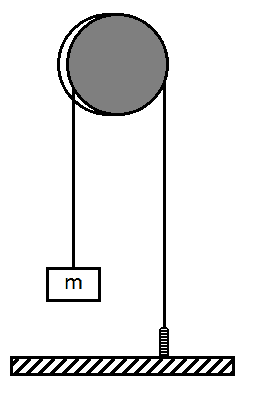# Spring - Pulley - Mass <Oscilations problem>

We have a mass M attached to a string.
In the other extreme of the string we have a spring.
We know the moment of inertia of the alloy I, and it's radius R.
We know the constant of the spring k.

The string is inextensible. String mass is negligible. There's not friction. There's no slipping. What's the frequence of the oscilation?

Attemp:
I know that the total energy in the system is:

$U = h g m + \frac{1}{2} I \omega ^{2} + \frac{1}{2} k x^{2}$
As the string is inextensible, we have $\Delta h = \Delta x \Leftrightarrow h-x = c$
Then, when h is at it's maximum x is at it's minimum. Also $\omega = 0$ at this time.
Then, by taking the following system of reference:
$0 = h_{min}$ and $0 = x_{max}$ we have that
$x_{min} = -h_{max}$
$\omega_{max}$ is reached when $h = \frac{h_{min}+h_{max}}{2} = \frac{h_{max}}{2}$. (Is this fine? How do I justify this? I'm guessing this movement is some of trigonometric function that's why I asserted that).
Then, as $\nu = \frac{h_{max}-h_{min}}{4 \pi} \frac{1}{\omega_{max} R}$
Due to the fact that $(a sin(b t))'= ab \frac{\nu}{2 \pi} cos(b t)$, then the maximum less the minimum of one, divided by the other gives us $\nu$

Now, as in our system of references we have:
$U = h g m$ when $h=h_{max}$ and when $h \frac{h_{max}}{2}$ we have:
$U = \frac{h_{max}}{2} g m + \big( \frac{1}{2} \frac{h_{max}}{2}\big) ^{2} k + \frac{1}{2} I \omega^{2}$
Then $\frac{h_{max}}{2} gm - \big( \frac{1}{2} \frac{h_{max}}{2}\big)^{2} k = \frac{1}{2} I \omega^{2}$
$\frac{h_{max}}{2} (gm-\frac{h_{max}}{4}) = \frac{1}{2} I \omega^{2}$
$\sqrt{\frac{h_{max}}{I} (gm-\frac{h_{max}}{4})} = \omega$
Then
$\nu = \dfrac{h_{max}}{4 \pi R \sqrt{\frac{h_{max}}{I} (gm-\frac{h_{max}}{4})} }$
I recently noticed that $h_{max}$ isn't given.
How do I deduct it?

PD: Forgive the bad drawing, I'm not very good with sketches.

#### Attachments

•Image.png
1 KB · Views: 498
Last edited:

SammyS
Staff Emeritus
Homework Helper
Gold Member
We have a mass M attached to a string.
In the other extreme of the string we have a spring.
We know the moment of inertia of the alloy I, and it's radius R.
We know the constant of the spring k.

The string is inextensible. String mass is negligible. There's not friction. There's no slipping. What's the frequence of the oscilation?

Attemp:
I know that the total energy in the system is:

$U = h g m + \frac{1}{2} I \omega ^{2} + \frac{1}{2} k x^{2}$
As the string is inextensible, we have $\Delta h = \Delta x \Leftrightarrow h-x = c$
Then, when h is at it's maximum x is at it's minimum. Also $\omega = 0$ at this time.
Then, by taking the following system of reference:
$0 = h_{min}$ and $0 = x_{max}$ we have that
$x_{min} = -h_{max}$
$\omega_{max}$ is reached when $h = \frac{h_{min}+h_{max}}{2} = \frac{h_{max}}{2}$. (Is this fine? How do I justify this? I'm guessing this movement is some of trigonometric function that's why I asserted that).
Then, as $\nu = \frac{h_{max}-h_{min}}{4 \pi} \frac{1}{\omega_{max} R}$
Due to the fact that $(a sin(b t))'= ab \frac{\nu}{2 \pi} cos(b t)$, then the maximum less the minimum of one, divided by the other gives us $\nu$

Now, as in our system of references we have:
$U = h g m$ when $h=h_{max}$ and when $h \frac{h_{max}}{2}$ we have:
$U = \frac{h_{max}}{2} g m + \big( \frac{1}{2} \frac{h_{max}}{2}\big) ^{2} k + \frac{1}{2} I \omega^{2}$
Then $\frac{h_{max}}{2} gm - \big( \frac{1}{2} \frac{h_{max}}{2}\big)^{2} k = \frac{1}{2} I \omega^{2}$
$\frac{h_{max}}{2} (gm-\frac{h_{max}}{4}) = \frac{1}{2} I \omega^{2}$
$\sqrt{\frac{h_{max}}{I} (gm-\frac{h_{max}}{4})} = \omega$
Then
$\nu = \dfrac{h_{max}}{4 \pi R \sqrt{\frac{h_{max}}{I} (gm-\frac{h_{max}}{4})} }$
I recently noticed that $h_{max}$ isn't given.
How do I deduct it?

PD: Forgive the bad drawing, I'm not very good with sketches.
Don't forget the kinetic energy of the mass.Thanks!
Then... it's something like this?
$U = \frac{h_{max}}{2} g m + \big( \frac{1}{2} \frac{h_{max}}{2}\big) ^{2} k + \frac{1}{2} I \omega^{2} + \frac{1}{2} m R^{2} \omega^{2}$
$\frac{h_{max}}{2} gm - \big( \frac{1}{2} \frac{h_{max}}{2}\big) ^{2} k = (\frac{1}{2} I + \frac{1}{2} m R^{2}) \omega^{2}$
$\dfrac{h_{max} ( gm - \frac{h_{max}^{2}}{4} k)}{I+mR^{2}} = ( I + m R^{2}) \omega^{2}$
$\sqrt{\dfrac{h_{max} ( gm - \frac{h_{max}^{2}}{4} k)}{I+mR^{2}}} = \omega$

$\nu = \frac{h_{max}}{4 \pi} \sqrt{\dfrac{I+mR^{2}}{h_{max} ( gm - \frac{h_{max}^{2}}{4} k)} } = \frac{1}{4 \pi} \sqrt{\dfrac{h_{max}(I+mR^{2})}{ gm - \frac{h_{max}^{2}}{4} k} }$

SammyS
Staff Emeritus
Homework Helper
Gold Member
It's the following expression which needs the kinetic energy of the mass added.
$U = h g m + \frac{1}{2} I \omega ^{2} + \frac{1}{2} k x^{2}$

In the end, the frequency is independent of hmax.

You really need to define all of your variables.

Is there a particular reason to do the problem using energy rather than force?

I tought it would be easier, only that =/ but now I think energy isn't the right approach.

Orodruin
Staff Emeritus